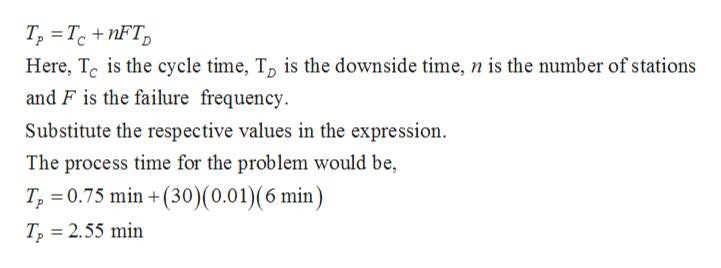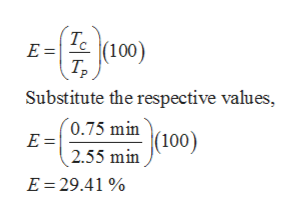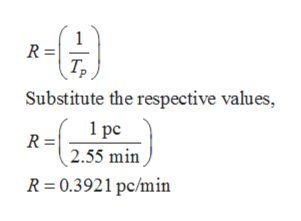# A 30-station transfer line has an ideal cycle time of 0.75 min, an average downtime of 6.0 min per line stop occurrence, and a station failure frequency of 0.01 for all stations. A proposal has been submitted to locate a storage buffer between stations 15 and 16 to improve line efficiency.(a) Determine the current line efficiency and production rate(b)Determine the maximum possible line efficiency and production rate that would result from the new proposal.(c)Consider a new proposal to divide the line into three stages: two storage buffers located between stations 10 and 11, and between stations 20 and 21, respectively.Answer Part (b) for this proposal.(d) Now consider the original proposal. Assume that the capacity of the proposed storage buffer is to be 20 parts, and the downtime (Td = 6.0 min) is a constant.Answer Part (b) for this proposal.

Question

A 30-station transfer line has an ideal cycle time of 0.75 min, an average downtime of 6.0 min per line stop occurrence, and a station failure frequency of 0.01 for all stations. A proposal has been submitted to locate a storage buffer between stations 15 and 16 to improve line efficiency.

(a) Determine the current line efficiency and production rate

(b)Determine the maximum possible line efficiency and production rate that would result from the new proposal.

(c)Consider a new proposal to divide the line into three stages: two storage buffers located between stations 10 and 11, and between stations 20 and 21, respectively.

Answer Part (b) for this proposal.

(d) Now consider the original proposal. Assume that the capacity of the proposed storage buffer is to be 20 parts, and the downtime (Td = 6.0 min) is a constant.

Answer Part (b) for this proposal.

check_circle

Step 1

a)

The expression of the process time for the transfer line having n stations is,help_outlineImage TranscriptioncloseT, TcnFT Here, Tc is the cycle time, To is the downside time, n is the number of stations and F is the failure frequency Substitute the respective values in the expression The process time for the problem would be, T 0.75 min +(30) (0.01)(6 min) T2 2.55 min fullscreen
Step 2

The current line efficiency would be,help_outlineImage TranscriptioncloseTc (100) E = Substitute the respective values, (0.75 min (100) E = 2.55 min E 29.41 fullscreen
Step 3

The current production...help_outlineImage Transcriptionclose1 R = Т, Substitute the respective values, 1 ре R = 2.55 min R 0.3921 pc/min fullscreen

### Want to see the full answer?

See Solution

#### Want to see this answer and more?

Solutions are written by subject experts who are available 24/7. Questions are typically answered within 1 hour.*

See Solution
*Response times may vary by subject and question.
Tagged in

### Mechanical Engineering